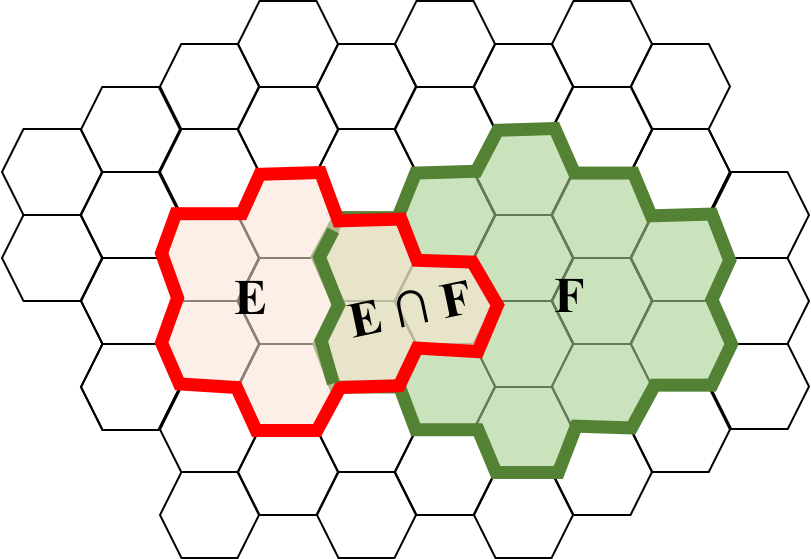# Law of Total Probability

An astute person once observed that when looking at a picture, like the one we saw for conditional probability:that event $E$ can be thought of as having two parts, the part that is in $F$, $(E \and F)$, and the part that isn’t, $(E \and F\c)$. This is true because $F$ and $F\c$ are (a) mutually exclusive sets of outcomes which (b) together cover the entire sample space. After further investigation this proved to be mathematically true, and there was much rejoicing:

$$\p(E) = \p(E \and F) + \p(E \and F\c)$$

This observation proved to be particularly useful when it was combined with the chain rule and gave rise to a tool so useful, it was given the big name, law of total probability.

The Law of Total Probability
If we combine our above observation with the chain rule, we get a very useful formula: $$\p(E) = \p(E | F) \p(F) + \p(E | F\c) \p(F\c)$$

There is a more general version of the rule. If you can divide your sample space into any number of mutually exclusive events: $B_1, B_2, \dots B_n$ such that every outcome in the sample space falls into one of those events, then: \begin{align} \p(E) &= \sum_{i=1}^n \p(E \and B_i) && \text{Extension of our observation}\\ &= \sum_{i=1}^n \p(E | B_i) \p(B_i) && \text{Using chain rule on each term} \end{align}

We can build intuition for the general version of the law of total probability in a similar way. If we can divide a sample space into a set of several mutually exclusive sets (where the $\or$ of all the sets covers the entire sample space) then any event can be solved for by thinking of the likelihood of the event and each of the mutually exclusive sets.In the image above, you could compute $\p(E)$ to be equal to $\p\Big[(E \and B_1) \text{ }\or \text{ }(E \and B_2) \dots\big]$. Of course this is worth mentioning because there are many real world cases where the sample space can be discretized into several mutual exclusive events. As an example, if you were thinking about the probability of the location of an object on earth, you could discretize the area over which you are tracking into a grid.